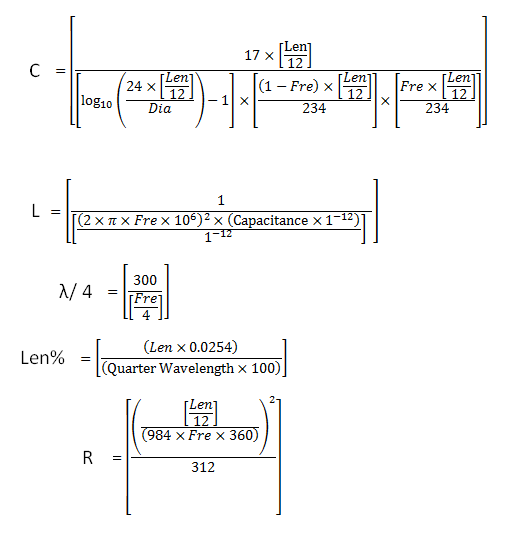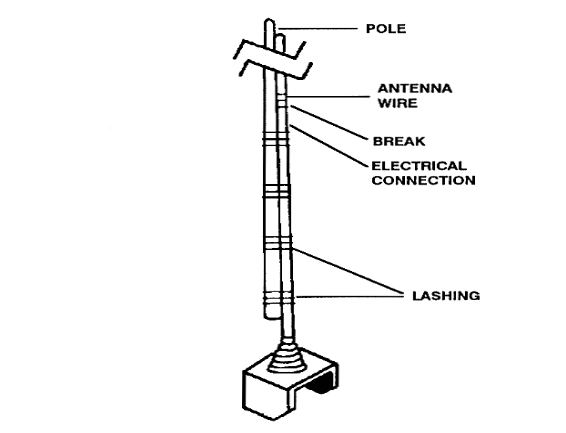# Whip Antenna Calculator

This CalcTown calculator calculates the various parameters related to the design of a Whip Antenna.

An antenna with single straight rod is the whip antenna. This is a calculator yields capacitance, tuning inductance, wavelength, length and radiation resistance.

#### Result

pF
nH
m
%
ΩWhere,

Fre = frequency

Dia = Diameter

Len = Length

C = Capacitance of the antenna

L = Inductance of the antenna

Len% = Length of the antenna in percentage

λ = Quarter Wavelength